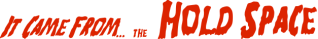Next40

# Fractional arithmetic without shell

• You can do fractional arithmetic in the shell

• Two insights are required

• The main one is to use rational arithmetic instead of floating-point

• Consider 3.45

• "3.45" won't work

• But 3.45 = 69/20, which will work

```          x_n=69;  x_d=20;
```

 NextCopyright © 2011 M. J. Dominus